## 15 Questions MCQ Test Quantitative Aptitude for SSC CGL | Test: Quadratic Equations- 1

Description
Attempt Test: Quadratic Equations- 1 | 15 questions in 30 minutes | Mock test for Quant preparation | Free important questions MCQ to study Quantitative Aptitude for SSC CGL for Quant Exam | Download free PDF with solutions
QUESTION: 1

### What is the value of x in the equation x2 + 9 = 6x ?

Solution:

Given, x2 + 9 = 6x

⇒ x2 - 6x + 9 = 0

⇒ x2 -3x -3x + 9 = 0

⇒ x ( x - 3) - 3 ( x - 3) = 0

⇒ (x - 3) (x - 3) = 0

⇒ x = 3

QUESTION: 2

### If the sum of three consecutive terms of an increasing A.P. is 51 and the product of the first and third of these terms is 273, then the third term is

Solution:

Let 3 consecutive terms A.P is a –d, a , a + d. and the sum is 51

so, (a –d) + a + (a + d) = 51
⇒ 3a –d + d = 51
⇒ 3a = 51
⇒ a = 17

The product of first and third terms is 273

So  it stand for ( a –d) (a + d) = 273
⇒ a2 –d2 = 273
⇒ 172 –d 2 = 273
⇒ 289 –d 2 = 273
⇒  d 2 = 289 –273
⇒ d 2 = 16
⇒ d = 4

Hence the 3rd terms ( a+d ) is 21

QUESTION: 3

### What is the number of integral solutions of the equation 2x2 – 3x – 2 = 0?

Solution:
QUESTION: 4

What is the value of s+t if the equation 3x2 + tx + s has 2 and 3 as its roots?

Solution:
QUESTION: 5

What is the sum of all values of x that satisfies the equation: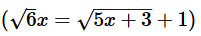Solution:

Explanation : By picking from the options, we can check which value satisfies the equation

For x = 28

√168 = √143+1

12.9 = (11.9+1)

12.9 = 12.9

QUESTION: 6

If integers p, q and r are the roots of the equation x3-7x2+12x = 0, and p < q < r, what is the value of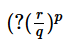?

Solution:

Step 1: Question statement and Inferences

Given equation is x3−7x2+12x=0

and p, q, r are the roots of this equation.

We need to find the value of the expression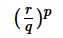. For this we need to find the values of p, q and r by solving the given equation.

Step 2 & 3: Finding required values and calculating the final answer

x3−7x2+12x=0

⇒x(x2−7x+12)=0 ....(I)

Note that x2−7x+12

is a standard form quadratic equation. It can be factorized as follows:

x2−4x−3x+12=(x−3)(x−4)

Therefore (I) can be rewritten as:

x(x-3)(x-4) = 0

By putting each factor equal to zero, we can find the roots of (I):

x = 0(Root 1)

x – 3 = 0

⇒ x = 3 (Root 2)

x – 4 = 0

⇒ x = 4 (Root 3)

Therefore possible values for p, q, r: {0, 3, 4}

Since it is given that p < q < r, we have:

p = 0, q = 3 and r =4

Therefore=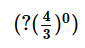When a number is raised to the power zero, we get 1.

So,=1=1

QUESTION: 7

If twice the reciprocal of x is equal to the sum of x and 1, how many values of x are possible?

Solution:

Step 1: Set up the equation based on the description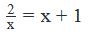Step 2 & 3: Finding required values and calculating the final answer

This is a quadratic equation, so x has either one or two possible answers (Every quadratic equation has two roots but variable x may have some constraints imposed on it, which make one of the roots an impossible value to have for x)

The constraint on x in the given equation is that x cannot be equal to zero (since x is in the denominator).

To find out how many values of x are possible, we set up the equation to find the roots.

First, multiply both sides by x to get rid of the fraction:

2=x2+x

Next, set the equation equal to zero:

x2+x−2=0

Finally, factor out the expressions:

(x+2)(x−1)=0

x has two possible values: -2 and 1,

QUESTION: 8

If (x−3)(2x−5)=0  and   (x−5/2)(x−4)=0, x = ?

Solution:

Step 1: Question statement and Inferences

We are given two quadratic equations in x. We will solve both of them and look for a common value of x

Step 2 & 3: Finding required values and calculating the final answer

Approach:

1. We observe that both the given quadratic equations are already in factored form.
2. By putting each factor of an equation equal to zero, we can find the roots of an equation. So, we will be able to find the two roots of each equation.
3. The common root of both equations will be our answer

Implementing the approach:

Finding the roots of the first equation:

(x - 3)(2x - 5)

Either

x - 3 = 0

• x = 3  . . . Root 1A

Or

2x - 5 = 0

• x = 5/2 . . . Root1B

Finding the roots of the second equation: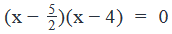Either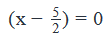• x = 5/2 . . . Root 2A

Or

x - 4 = 0

• x = 4 . . . Root 2B

We observe that x=5/2

is a common root of both equations.

• The value of x that satisfies both equations is x=5/2

QUESTION: 9

if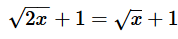and x is not a positive integer, x = ?

Solution:

Step 1: Question statement and Inferences

Given that x is not a positive integer and we need to find the value of x from the given equation.

Step 2 & 3: Finding required values and calculating the final answer

Given equation is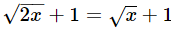Squaring on both sides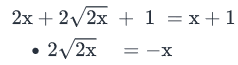​Squaring on both sides

8x  =x2

• x2 -8x=0
• x(x -8)=0

Putting each factor to zero, we get:

x = 0 or x = 8

However, since it is already given that x is not a positive integer, x≠8.

Therefore x = 0.

QUESTION: 10

If x >0, what is the value of x?

(1)   x is equal to half of its square root

(2)   4x2 + 7x – 2 = 0

Solution:

Steps 1 & 2: Understand Question and Draw Inferences

We are given that x is a positive integer

• x > 0

Step 3: Analyze Statement 1

Writing the information given in statement 1 in an equation form:

x=(√x)/2

Squaring both sides:

x2=x/4

x2−x/4=0  (a standard form Quadratic equation)

x(x−1/4)=0

Now that the equation is in factorized form, we can find the roots.

Either x = 0

Or,

x–1/4=0

x=1/4

Thus, two values of x can satisfy the given equation.

However, we are given that x > 0

• 0 cannot be a value of x.

This means that  x=1/4

Thus, a unique value of x is obtained from the information given in Statement 1

SUFFICIENT

Step 4: Analyze Statement 2

We are given a quadratic equation in the standard form:

4x2 + 7x – 2 = 0

We will factorize this equation to find its roots:

4x2 + 8x – x – 2 = 0

• 4x(x + 2) -1 (x+2) = 0
• (4x-1)(x+2) = 0

Now that the given equation has been factorized, we can find the roots by putting each factor equal to zero.

Either

4x – 1 = 0

x=1/4

Or

x + 2 = 0

x = -2

Given: x > 0

Therefore, x cannot have the value -2.

Thus, x=1/4

A unique value of x is obtained from the information given in Statement 2.

SUFFICIENT

Step 5: Analyze Both Statements Together (if needed)

We get a unique answer in steps 3 and4, so this step is not required

QUESTION: 11

If x + y = -10 and xy = 16, which of the following statements must be true?

(I) x – y = -6

(II) x2 + y2 = 68

(III) (x-y)2 leaves a remainder of 1 when divided by 7

Solution:

Step 1: Question statement and Inferences

It is given that

x+y=−10 ....(i)

xy=16  ....(ii)

We need to find which of the given statements must be true.

Step 2 & 3: Finding required values and calculating the final answer

Squaring (i) on both sides, we get:

(x+y)2=100

x2+y2+2xy=100

....(iii)

We know that

(x−y)2=x2+y2−2xy

(x−y)2=x2+y2+2xy−4xy

(x−y)2=(x+y)2−4xy

Using (ii) and (iii) we get:

(x−y)2=100−64

(x−y)2=36

⇒(x−y)=±6

Therefore (I) is not a must be true statement.

Now let us look at (II).

(iii) – 2*(ii) gives us:

(x2+y2+2xy)−2xy=100−32

⇒x2+y2=68

Therefore statement II is true.

(x−y)2=36

We know that

36=35+136=7∗5+1

Therefore

(x−y)2

leaves a remainder of 1 when divided by 7.

QUESTION: 12

When a body falls freely under gravity, the distance d covered by it in time t is given by the formula:​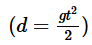, where g is a constant called acceleration due to gravity.

When a ball is dropped from a 16-feet high structure on earth, it takes 1 second to reach the ground. How much time will it take for a ball to reach the ground if it is dropped from a height of 2800 feet on a planet whose acceleration due to gravity is seven times that of earth?

Solution:

Step 1: Question statement and Inferences

We are given an equation to calculate the distance travelled (d) by a free falling body within a time (t)....(I)

It is given that on earth, a ball dropped from a height of 16 feet takes 1 second to reach the ground.

Therefore from (I), we have

16=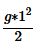⇒g=32

Thus, we have found the value of acceleration due to gravity for earth.

Let the acceleration due to gravity of the other planet be GP

It is given that GP=7g

⇒GP=7*32……. (II)

Step 2 & 3: Finding required values and calculating the final answer

Once again, using (I) we can write the equation for the free-fall of the ball on the other planet:

2800=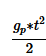However, from (II), we know that

GP=7*32

Using this in the above equation, we get,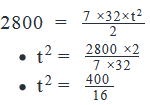•
• t2= 25
• t2 – 25 = 0
• (t -5)(t + 5) = 0
• t = 5 or t = -5

However, t denotes time. Therefore, it cannot have a negative value.

Rejecting the negative value, we get t = 5

Thus, on the other planet, it will take a ball 5 seconds to reach the ground from a height of 2800 feet under conditions of free fall.

QUESTION: 13

Given the equation x2 + bx + c = 0, where b and c are constants, what is the value of c?

(1)   The sum of the roots of the equation is zero.

(2)   The sum of the squares of the roots of the equation is equal to 18.

Solution:

Steps 1 & 2: Understand Question and Draw Inferences

Given equation is

x2+bx+c=0 …………….. (I)

Let us suppose that the roots of this equation are p and q.

Given that b and c are constants, we need to find the value of c.

We know that for a quadratic equation

ax2+bx+c=0

Sum of roots = −b/a

Product of roots = c/a

In the given equation, a = 1

Therefore,

Sum of roots = -b

Product of roots = c

Step 3: Analyze Statement 1

It is given that the sum of roots of (I) is zero.

So, we have:

-b = p + q = 0

• b = 0 ………….. (II)

We cannot find the value of c from this information.

Statement 1 alone is not sufficient to arrive at a unique answer.

Step 4: Analyze Statement 2

Now let us look at statement 2.

Writing it in equation form, we have:

p2+q2=18

We know that

p2+q2=(p+q)2−2pq

• p2+q2=(−b)2−2c
• (−b)2−2c=18
• b2−2c=18 ………….. (III)

But we need the value of b here to find the value of c.

Statement 2 alone is not sufficient to arrive at a unique answer.

Step 5: Analyze Both Statements Together (if needed)

Now, let us look at both the statements together.

From statement 1, we have

b = 0

From statement 2, we have

b- 2c = 18

Combining both of these, we get:

-2c = 18

• c = -9

QUESTION: 14

If x is an integer and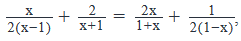what is the value of x?

Solution:

Given equation is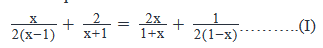And, we need to find the value of x, given that x is an integer.

Step 2 & 3: Finding required values and calculating the final answer

Let us rewriting the last term of (I) to get x-1 in the denominator: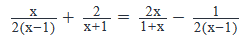Let us now bring the terms with the same denominator together: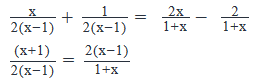• (x + 1)2 = {2(x – 1)}2
• [(x+1)2-{2(x-1)}2 ]=0 ………….. (II)
• Observe that this is of the form  a2 – b2 = (a+b)(a-b)

So let us say:

a = x + 1

b = 2(x – 1) = 2x - 2

Therefore:

a + b = x + 1 + 2x – 2 = 3x -1

a – b = x + 1 - 2x + 2 = -x + 3

Therefore we can rewrite (II) as:

(3x-1)(3-x)=0

• Putting each factor to zero: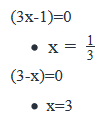Since it is given that x is an integer,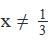Thus, x = 3 is the required answer.

QUESTION: 15

In the equation ax2 + bx + c = 0, where a, b and c are constants and a ≠ 0, what is the value of b?

(1)    3 and 4 are roots of the equation.

(2)   The product of the roots of the equation is 12.

Solution:

Steps 1 & 2: Understand Question and Draw Inferences

Given equation is

ax2+bx+c=0

It is also given that a, b and c are constants and that a≠0.

We need to find the value of b.

Step 3: Analyze Statement 1

It is given that 3 and 4 are the roots of the equation.

We know that for a quadratic equation
ax2+bx+c=0

Sum of roots = −b/a

Product of roots = c/a

Therefore, we have:

−ba  = 3 + 4 = 7………..(I)

c/ a  = 3 * 4 = 12 …………(II)

Note that these are two linear equations in three variables. So we cannot solve exclusively for b.

Statement 1 alone is not sufficient to arrive at a unique answer.

Step 4: Analyze Statement 2

It is given that the product of roots is 12.

Therefore, we have:

c/a  = 12 …….(III)

Notice that (III) is a single linear equation in two variables. Moreover, it doesn’t provide any information about b.

So statement 2 alone is not sufficient to arrive at a unique answer.

Step 5: Analyze Both Statements Together (if needed)

Now let us look at both the statements together.

Statement 1 gives us (I) and (II)

Statement 2 gives us (III).

However, notice that (III) is essentially same as (II).

In other words, we are simply left with (I) and (II) (essentially the same situation as in statement 1).

Therefore statement 1 and statement 2 combined together are not sufficient to arrive at a unique answer.Use Code STAYHOME200 and get INR 200 additional OFF Use Coupon Code